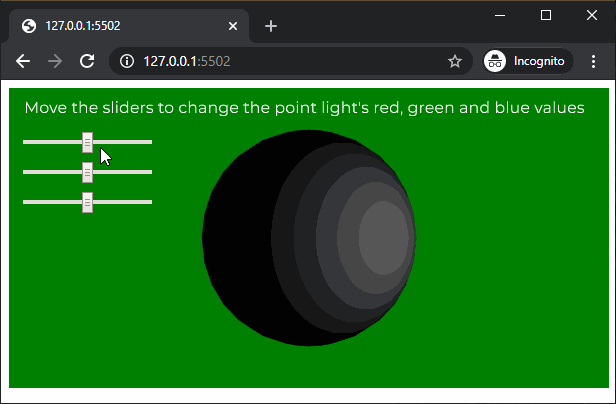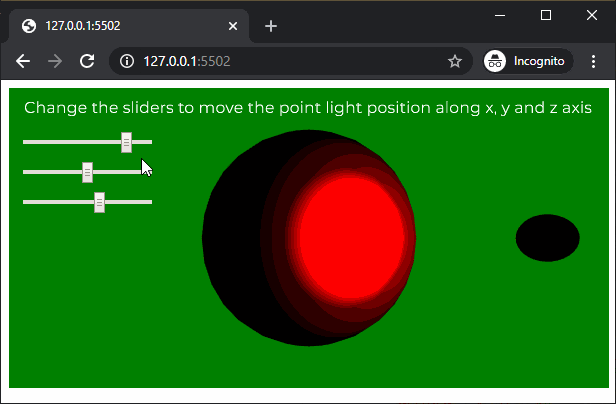# p5.js | pointLight() Function

• Last Updated : 27 Apr, 2020

The pointLight() function in p5.js is used to create a point lights with the specified color and position in the scene. A scene can have a maximum of 5 active point lights at a time.

Syntax:

`pointLight( v1, v2, v3, x, y, z )`

OR

`pointLight( v1, v2, v3, position )`

OR

`pointLight( color, x, y, z )`

OR

`pointLight( color, position )`

Parameters: This function accept eight parameters as mentioned above and described below:

• v1: It is a number which determines the red or hue value relative to the current color range.
• v2: It is a number which determines the green or saturation value relative to the current color range.
• v3: It is a number which determines the blue or brightness value relative to the current color range.
• x: It is a number which determines the x axis position of the light.
• y: It is a number which determines the y axis position of the light.
• z: It is a number which determines the z axis position of the light.
• position: It is p5.Vector which defines the position of the light.
• color: It is a color string or p5.Color which defines the color of the point light.

Below example illustrates the pointLight() function in p5.js:

Example 1:

 `let newFont; ` `let directionalLightEnable = ``false``; ` ` `  `function` `preload() { ` `  ``newFont = loadFont(``'fonts/Montserrat.otf'``); ` `} ` ` `  `function` `setup() { ` `  ``createCanvas(600, 300, WEBGL); ` `  ``textFont(newFont, 16); ` ` `  `  ``redSlider = createSlider(0, 255, 128, 1); ` `  ``redSlider.position(20, 50); ` ` `  `  ``greenSlider = createSlider(0, 255, 128, 1); ` `  ``greenSlider.position(20, 80); ` ` `  `  ``blueSlider = createSlider(0, 255, 128, 1); ` `  ``blueSlider.position(20, 110); ` `} ` ` `  `function` `draw() { ` `  ``background(``'green'``); ` `  ``text(``"Move the sliders to change the point light's red, "``+ ` `                        ``"green and blue values"``, -285, -125); ` `  ``noStroke(); ` ` `  `  ``redValue = redSlider.value(); ` `  ``greenValue = greenSlider.value(); ` `  ``blueValue = blueSlider.value(); ` ` `  `  ``// Create a point light with the selected light color ` `  ``pointLight(redValue, greenValue, blueValue, 100, 0, 150); ` ` `  `  ``ambientMaterial(255); ` ` `  `  ``// Create the sphere on which the point light will work ` `  ``sphere(100); ` `} `

Output:Example 2:

 `let newFont; ` `let directionalLightEnable = ``false``; ` ` `  `function` `preload() { ` `  ``newFont = loadFont(``'fonts/Montserrat.otf'``); ` `} ` ` `  `function` `setup() { ` `  ``createCanvas(600, 300, WEBGL); ` `  ``textFont(newFont, 16); ` ` `  `  ``xPosSlider = createSlider(-150, 150, 100, 1); ` `  ``xPosSlider.position(20, 50); ` ` `  `  ``yPosSlider = createSlider(-300, 300, 0, 1); ` `  ``yPosSlider.position(20, 80); ` ` `  `  ``zPosSlider = createSlider(0, 250, 150, 1); ` `  ``zPosSlider.position(20, 110); ` ` `  `} ` ` `  `function` `draw() { ` `  ``background(``'green'``); ` `  ``text(``"Change the sliders to move the point light position "``+ ` `                          ``"along x, y and z axis"``, -285, -125); ` `  ``noStroke(); ` ` `  `  ``xPositionValue = xPosSlider.value(); ` `  ``yPositionValue = yPosSlider.value(); ` `  ``zPositionValue = zPosSlider.value(); ` ` `  `  ``// Create a point light at the selected location ` `  ``pointLight(255, 0, 0, xPositionValue, yPositionValue, zPositionValue); ` ` `  `  ``// Create a sphere to show the demonstrate ` `  ``// of the point light location ` `  ``translate(xPositionValue, yPositionValue, zPositionValue); ` `  ``sphere(10); ` `  ``translate(-xPositionValue, -yPositionValue, -zPositionValue); ` ` `  `  ``specularMaterial(255); ` ` `  `  ``// Create the sphere on which the point light will work ` `  ``sphere(100); ` `} `

Output:Online editor: https://editor.p5js.org/

My Personal Notes arrow_drop_up
Recommended Articles
Page :